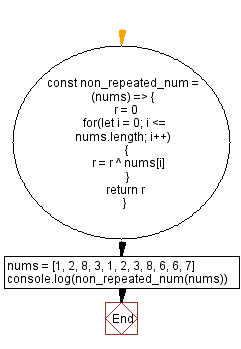# JavaScript - Find the non-repeated element from an array

## JavaScript Bit Manipulation: Exercise-14 with Solution

In an array every element appears twice except for one. Write a JavaScript program to find the non-repeated element in an array using bit manipulation.

Test Data:
() -> 1
([1, 2, 3]) -> 0 [All elements are non- repeated]
[1, 2, 8, 3, 1, 2, 3, 8, 6, 6, 7] -> 7

Sample Solution:

JavaScript Code:

``````const non_repeated_num = (nums) => {
r = 0
for(let i = 0; i <= nums.length; i++)
{
r = r ^ nums[i]
}
return r
}
nums = [1, 2, 8, 3, 1, 2, 3, 8, 6, 6, 7]
console.log(non_repeated_num(nums))
```
```

Sample Output:

```7
```

Flowchart:Live Demo:

See the Pen javascript-bit-manipulation-exercise-14 by w3resource (@w3resource) on CodePen.

* To run the code mouse over on Result panel and click on 'RERUN' button.*

Improve this sample solution and post your code through Disqus

What is the difficulty level of this exercise?

Test your Programming skills with w3resource's quiz.

﻿